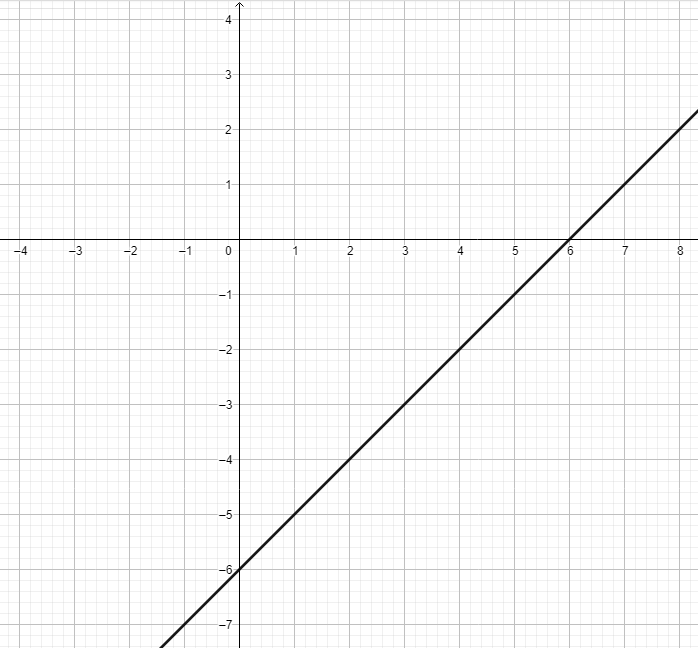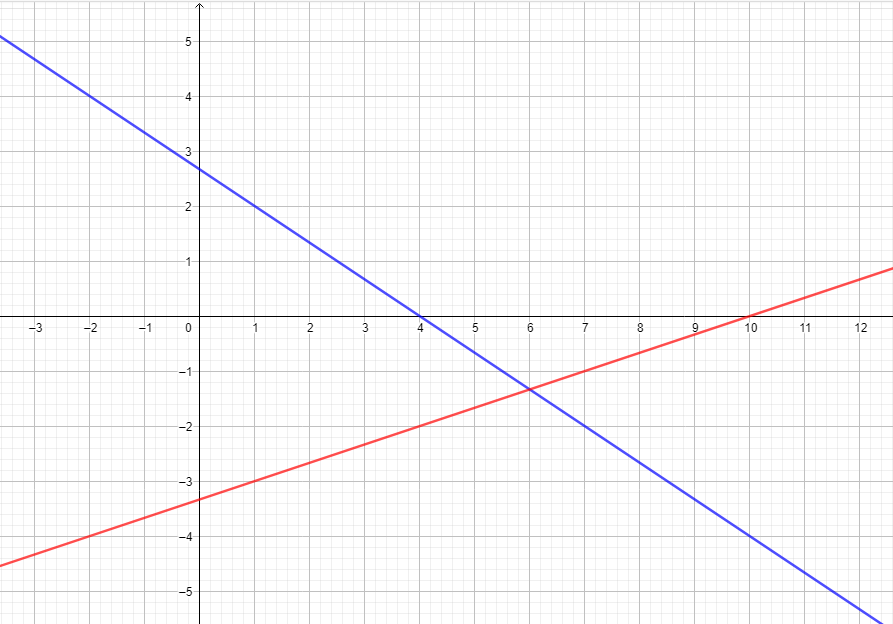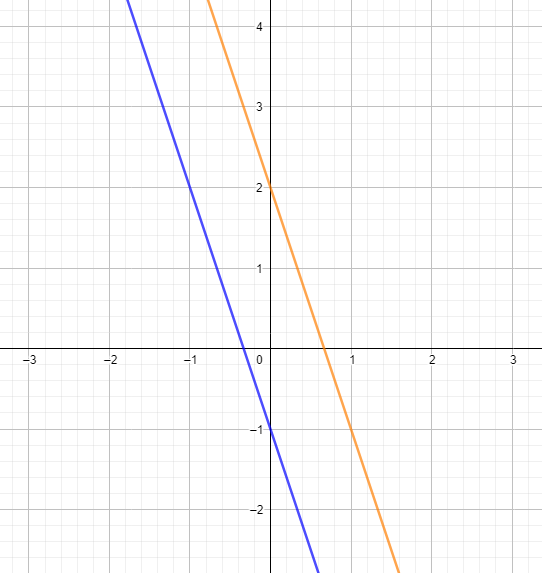# 8th Grade - Introduction to Systems of Equations

## Introduction

• Equations like $x-y=8$ and $3x+y=16$ are called simultaneous linear equations or a system of linear equations if our goal is to make them true for the same set of numbers.
• An ordered pair of real numbers that satisfy both equations is a solution to the system.
• This solution to the system can be determined using the graphical method or algebraic method.

## Types of Linear Systems in Two Variables

• Consistent and dependent equations - this is a system of linear equations having infinitely many solutions. The slopes and y-intercepts of the lines described by the equations are equal. A linear system $\left\{\begin{array}{l}{a}_{1}x+{b}_{1}y={c}_{1}\\ {a}_{2}x+{b}_{2}y={c}_{2}\end{array}\right\$ is consistent and dependent if $\frac{{a}_{1}}{{a}_{2}}=\frac{{b}_{1}}{{b}_{2}}=\frac{{c}_{1}}{{c}_{2}}$.
• Consistent and independent equations - this is a system of linear equations having exactly one solution. The slopes of the lines defined by the equations are not equal. The y-intercepts of the lines could be equal or unequal. A linear system $\left\{\begin{array}{l}{a}_{1}x+{b}_{1}y={c}_{1}\\ {a}_{2}x+{b}_{2}y={c}_{2}\end{array}\right\$ is consistent and independent if $\frac{{a}_{1}}{{a}_{2}}\ne \frac{{b}_{1}}{{b}_{2}}$.
• Inconsistent equations - this is a system of linear equations having no solution. The slopes of the lines defined by the equations are equal, but their y-intercepts are not equal. A linear system $\left\{\begin{array}{l}{a}_{1}x+{b}_{1}y={c}_{1}\\ {a}_{2}x+{b}_{2}y={c}_{2}\end{array}\right\$ is inconsistent if $\frac{{a}_{1}}{{a}_{2}}=\frac{{b}_{1}}{{b}_{2}}\ne \frac{{c}_{1}}{{c}_{2}}$.

## Graphs of Systems of Linear Equations

1. A linear system that has infinitely many solutions has coinciding graphs. The graph of $\left\{\begin{array}{l}3x-3y=18\\ x-y=6\end{array}\right\$ is shown below.2. A linear system with only one solution has a graph of intersecting lines. Consider the example of $\left\{\begin{array}{l}2x+3y=8\\ x-3y=10\end{array}\right\$.3. A linear system with no solution has a graph of parallel lines. The graph of $\left\{\begin{array}{l}3x+y=-1\\ 3x+y=2\end{array}\right\$ is shown below.## Solved Examples

Example 1. Solve the system $\left\{\begin{array}{l}3x+2y=6\\ -3x+3y=14\end{array}\right\$ using the elimination method.

Solution:

Eliminate $x$ by adding the two equations.

$\left(3x+2y=6\right)+\left(-3x+3y=14\right)$

$5y=20$

$⇒y=4$

Substitute the value of $y$ to any of the equations in the system.

$3x+2\left(4\right)=6$

$3x=6-8$

$3x=-2$

$⇒x=-\frac{2}{3}$

Example 2. Solve the system $\left\{\begin{array}{l}2x+y=4\\ -x+y=8\end{array}\right\$ using the substitution method.

Solution:

Use $-x+y=8$ to express $y$ in terms of $x$.

$-x+y=8\to y=x+8$

Substitute $\left(x+8\right)$ in the equation $2x+y=4$.

$2x+x+8=4$

$⇒x=-\frac{4}{3}$

$⇒y=\frac{20}{3}$

## Real-Life Situations involving Systems of Linear Equations in Two Variables

• There are many instances in the real-world context wherein we can apply the systems of linear equations in two variables. These situations involve two quantities that are related together, which are represented typically in symbols such as $x$ and $y$.
• The common areas of problem-solving involving systems of linear equations are Number-Related problems, Age Problems, Digit Problems, Work Problems, Distance-Speed-Time Problems, Measurement Problems, Mixture Problems, and Business-Related Problems.

Example:

A grocery store sells apples and oranges. On a certain day, 120 fruits are sold for a total of $156. If an apple costs$1.50 and orange costs \$2, what is the number of apples and oranges sold?

Solution:

Let $x$ be the number of apples sold and $y$ be the number of oranges sold.

The working equations are in the system $\left\{\begin{array}{l}x+y=120\\ 1.5x+2y=156\end{array}\right\$

Using any convenient method to solve these equations, we can find out that there are 72 apples and 48 oranges were sold on that day.

## Cheat Sheet

• The ordered pair $\left(x,y\right)$ is a solution to a linear system $\left\{\begin{array}{l}{a}_{1}x+{b}_{1}y={c}_{1}\\ {a}_{2}x+{b}_{2}y={c}_{2}\end{array}\right\$ if it satisfies both equations in the system. This implies that the values of x and y make the two equations in the system true.
• Consider the example of a linear system $\left\{\begin{array}{l}3x-2y=10\\ 4x+y=4\end{array}\right\$. This is a consistent and independent system of equations.
• Consider the example of a linear system $\left\{\begin{array}{l}7x-8y=-1\\ 14x-16y=12\end{array}\right\$. This is an inconsistent system of equations.
• Consider the example of a linear system $\left\{\begin{array}{l}5x-4y=2\\ 15x-12y-6=0\end{array}\right\$. This is a consistent and dependent system of equations.

## Blunder Areas

• Basic integer operations, properties of equality, and other algebraic procedures must be mastered to solve the system of linear equations efficiently.
• The equations in the linear system can be transformed to $y=mx+b$ to determine the type of solution they have.
• Not all equations in the linear system are given in the standard form $\left\{\begin{array}{l}{a}_{1}x+{b}_{1}y={c}_{1}\\ {a}_{2}x+{b}_{2}y={c}_{2}\end{array}\right\$. In cases like $\left\{\begin{array}{l}\frac{1}{2}x-y=2\\ x+\frac{2}{3}y=-1\end{array}\right\$, we need to simplify the equation first before finding the solution to the system.
• After finding the values of x and y, always check the values in both equations.